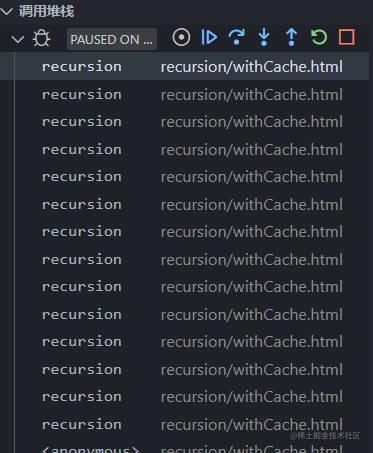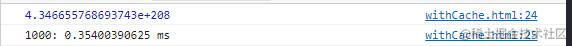# 递归与动态规划？简简单单明明白白的入门指南 ​

## 斐波那契数列 ​

1. 首先我们假设有一个函数能够计算出这个结果，这个函数输入一个参数n，代表第n位，函数的返回结果是数列第n位的值，函数取个名叫recursion，也就是`recursion(n)`
js
``````function recursion(n) {
return '数列第n位的值'
}``````
1. 数列要求每个数字都是前两位数的相加，n的前两位也就是n-1、n-2，他们相加要等于第n位的值，所以`recursion(n) = recursion(n-1) + recursion(n-2)`
js
``````function recursion(n) {
return recursion(n-1) + recursion(n-2)
}``````
1. 上面我们抽象出了数列的计算方法，当然我们知道这是有条件的，n必须大于等于3，因为小于3就没有前两位值了，又哪来的两个值相加。
js
``````function recursion(n) {
if ([1, 2].includes(n)) {
return 1
} else {
return recursion(n - 1) + recursion(n - 2)
}
}``````

recursion(3) recursion(5) recursion(10)都没有问题，当你recursion(100)，浏览器未响应了！！？？

js
``````console.time('10')
console.log(recursion(10))
console.timeEnd('10')

console.time('20')
console.log(recursion(20))
console.timeEnd('20')

console.time('30')
console.log(recursion(30))
console.timeEnd('30')

console.time('40')
console.log(recursion(40))
console.timeEnd('40')``````## 怎么解决 ​

js
``let cache = [null, 1, 1]``

js
``````if (cache.includes(n)) {
return cache[n]
}``````

js
``````else {
if (!cache[n - 1]) cache[n - 1] = recursion(n - 1)
if (!cache[n - 2]) cache[n - 2] = recursion(n - 2)
return cache[n - 1] + cache[n - 2]
}``````

js
``````let cache = [null, 1, 1]
function recursion(n) {
if (cache.includes(n)) {
return cache[n]
} else {
if (!cache[n - 2]) cache[n - 2] = recursion(n - 2)
if (!cache[n - 1]) cache[n - 1] = recursion(n - 1)
return cache[n - 1] + cache[n - 2]
}
}``````

js
``````console.time('1000')
console.log(recursion(1000))
console.timeEnd('1000')``````## 动态规划 ​

js
``````function dp(n) {
const arr = [1, 1]
for (let idx = 2; idx < n; idx++) {
arr[idx] = arr[idx - 1] + arr[idx - 2]
}
return arr.pop()
}``````

js
``````function dp(n) {
return new Array(n - 2).fill('').reduce((pre) => [...pre, pre[pre.length - 1] + pre[pre.length - 2]], [1, 1]).pop()
}``````

1. 首先，我们要先整理问题的状态方程，相当于我们例子中的`fn(n) = fn(n-1) + fn(n-2)`
2. 然后我们需要确认问题的边界条件，相当于例子中，n需要>2，n=1、n=2时结果都为1
3. 最后正向的计算结果值，直至获取到我们需要的结果